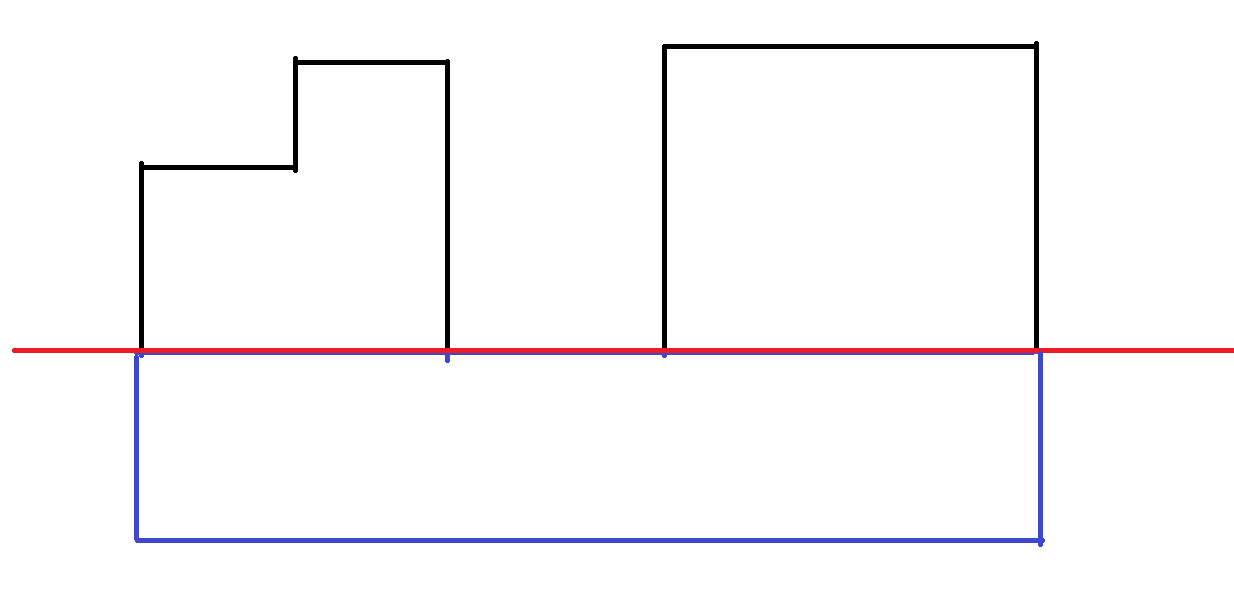## 0x01 签到题

input里面输key就行了，只不过限制了输入框长度。改HTML或者手动curl或用浏览器Dev Tool都可以。

HTTP ERROR 418。

## 0x0B 秘籍残篇

https://zb3.me/malbolge-tools/

（这题我也是实力傻逼）

## 0x10 FLXG的秘密

（我明明开过plain，然而没看末尾，实力眼瞎++）

## 0x12 加密算法和解密算法

（因为当时人懒，直接用的行列式求值，结果因为少加个等号调了半天）

（跑路跑路）

## [BZOJ2616][SPOJ PERIODNI] Periodni (树形背包)

BZOJ传送门

SPOJ传送门

### 数据范围

$n,m\leq500\ h_i\leq1000000$

## 题解$$dp_{i+j}=\sum dp_i\times C_{\Delta h}^j \times A_{len}^j$$
（似乎$dp_0$要特殊处理）

## 代码

#include<iostream>
#include<algorithm>
#include<cstring>
#include<cstdio>
#include<queue>
#include<bitset>
using namespace std;
template<typename __T>
{
x=0;
int f=1;char c=getchar();
while(!isdigit(c)){if(c=='-')   f=-1;c=getchar();}
while(isdigit(c))   {x=x*10+c-'0';c=getchar();}
x*=f;
}
const int mod=1000000007;
int frac;
int fracinv;
long long qpow(long long a,long long b)
{
long long ans=1;
while(b)
{
if(b&1) ans=(ans*a)%mod;
b>>=1;
a=a*a%mod;
}
return ans;
}
int C(int n,int m)
{
if(n<m) return 0;
return 1ll*frac[n]*fracinv[n-m]%mod*fracinv[m]%mod;
}
int Cinv(int n,int m)
{
return 1ll*fracinv[n]*frac[n-m]%mod*frac[m]%mod;
}
int A(int n,int m)
{
return 1ll*frac[n]*fracinv[n-m]%mod;
}
int h;
int n,k;
int dp;
int tmp;
int tot=1;
void dfs(int l,int r,int id,int lasth)
{
int minz=12345678;
for(int i=l;i<=r;i++)
minz=min(minz,h[i]);
int top=0;
int sta;
for(int i=l;i<=r;i++)
if(h[i]==minz)
sta[top++]=i;
if(top==r-l+1)
{
for(int i=0;i<=top;i++)
dp[id][i]=1ll*C(minz-lasth,i)*A(top,i)%mod;
return;
}
int laspos=l;
int edpos=l;
dp[id]=1;
while(laspos<=r)
{
while(h[laspos]==minz)  laspos++;
if(laspos>r)    break;
edpos=laspos;
while(edpos<r && h[edpos+1]>minz)   edpos++;
int neid=++tot;
dfs(laspos,edpos,neid,minz);
memcpy(tmp,dp[id],4*k+4);
memset(dp[id],0,4*k+4);
for(int i=0;i<=laspos;i++)
for(int j=0;j<=edpos-laspos+1;j++)
dp[id][i+j]=(dp[id][i+j]+1ll*tmp[i]*dp[neid][j])%mod;
dp[id]=1;
laspos=edpos+1;
}
memcpy(tmp,dp[id],4*k+4);
memset(dp[id],0,4*k+4);
for(int i=0;i<=r-l+1-top;i++)
for(int j=0;j<=r-l+1-i;j++)
dp[id][i+j]=(dp[id][i+j]+1ll*tmp[i]*C(minz-lasth,j)%mod*A(r-l+1-i,j))%mod;
dp[id]=1;
}
int main()
{
int sbsx=n;
for(int i=0;i<n;i++)
{
sbsx=max(sbsx,h[i]);
}
frac=1;
for(int i=1;i<=sbsx;i++)
frac[i]=1ll*frac[i-1]*i%mod;
fracinv[sbsx]=qpow(frac[sbsx],mod-2);
for(int i=sbsx-1;i>0;i--)
fracinv[i]=1ll*fracinv[i+1]*(i+1)%mod;
fracinv=1;
dfs(0,n-1,1,0);
printf("%d\n",dp[k]);
return 0;
}


AAA

0.0

Ctsc模拟赛By 洁妹

# 题解

$$E(x)=\frac{P(i-1)\times E(i-1)+P(i+1)\times E(i+1)}{P(x-1)+P(x+1)}+\frac{T}{2x(n-x)}\\=\frac{(x-1)E(x-1)+(x+1)E(x+1)}{2x}+\frac{n(n-1)}{2x(n-x)}$$

$$\frac{f(x)\times n}{x}=\frac{f(x-1)\times n+f(x+1)\times n}{2x}+\frac{n(n-1)}{2x(n-x)}\\ 2f(x)=f(x-1)+f(x+1)+\frac{n-1}{n-x}\\ f(x+1)=2f(x)-f(x-1)-\frac{n-1}{n-x}$$

（下面的结论我是先找规律后证明的，过程较乱）

$$f(n)=2f(n-1)-f(n-2)-(n-1)=0$$

$$(n-x+1)f(x)-(n-x)f(x-1)-(n-x)(n-1)=0$$

$$2(n-x+1)f(x-1)-(n-x+1)f(x-2)-(n-1)\\-(n-x)f(x-1)-(n-x)(n-1)\\ =(n-x+2)f(x-1)-(n-x-1)f(x-2)\\-(n-x+1)(n-1)=0$$

$$(n-X+1)f(X)-(n-X)f(X-1)-(n-X)(n-1)=0$$

$$nf(1)-(n-1)f(0)-(n-1)(n-1)=0\\ f(1)=\frac{(n-1)^2}{n}$$

# 代码

#include<iostream>
#include<cstdio>
#include<cstring>
#include<algorithm>
#include<queue>
#include<set>
using namespace std;
template<typename __T>
{
x=0;
int f=1;char c=getchar();
while(!isdigit(c)){if(c=='-')   f=-1;c=getchar();}
while(isdigit(c))   {x=x*10+c-'0';c=getchar();}
x*=f;
}
char str;
int cnt;
double dp;
int n;
int main()
{
scanf("%s",str);
n=strlen(str);
for(int i=0;i<n;i++)
cnt[str[i]-'A']++;
dp=(n-1.0)*(n-1.0)/n;
for(int i=1;i<n-1;i++)
dp[i+1]=2*dp[i]-dp[i-1]-(n-1.0)/(n-i);
double ans=0;
for(int i=0;i<26;i++)
ans+=dp[cnt[i]];
printf("%.1lf\\n",ans);
return 0;
}



liu-runda

CQZhangyu

miskcoo

rising_shit